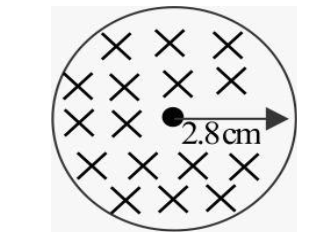Courses
Courses for Kids
Free study material
Free LIVE classes
More# The magnetic field of a cylindrical magnet that has a pole face radius 2.8cm can be varied sinusoidally between the minimum value of 16.8T and the maximum value of 17.2T at a frequency of $\dfrac{{50}}{\pi }Hz$.Cross section of the magnetic field created by the magnet is shown in figure. At a radial distance of 2cm from the axis, find the amplitude of the electric field(in units of $\mathop { \times 10}\nolimits^{ - 2} \mathop {mNC}\nolimits^{ - 1}$) induced by the magnetic field variation.Verified
290.4k+ views
HINT the increment of the magnetic field induces an emf to the electron placed in the field.this induced emf is due to change in flux. The magnetic flux is a product of magnetic field and area of cross section. We can assign this to the induced emf equation to find the acceleration.

Complete step by step solution
According to question we have given that,
Minimum value=16.8T
Maximum value=17.2T
Frequency=$\dfrac{{50}}{\pi }Hz$
We have to find the amplitude of the electric field induced by the magnetic field variation.
Firstly we can find the change in magnetic field$\left( {\Delta B} \right) =$Maximum value-Minimum value
Therefore, $\Delta B = 17.2 - 16.8 = 0.4T$
We know that the Time period$\left( t \right) = \dfrac{1}{\upsilon }$
Thus, $\dfrac{{\Delta B}}{t} = 0.4 \times \dfrac{{50}}{\pi } = \dfrac{{20}}{\pi }T/s$
The induced emf is given by faraday’s law as:
$e = - \dfrac{{d\phi }}{{dt}}$
and the induced emf can be written in terms of electric field as:
$e = \smallint \vec E.d\vec s$
Thus $\smallint \vec E.d\vec s = - \dfrac{{d\phi }}{{dt}}$
The flux equal to the magnetic field multiplied with the area, where the area is constant and the magnetic field is a function of time, so:
Using this relation,
$\smallint \vec E.d\vec t = \vec A.\dfrac{{d\vec B}}{{dt}}$
For, $r < R$
$E \times 2\pi r = \mathop {\pi r}\nolimits^2 \times \dfrac{{\Delta B}}{t}$
$\Rightarrow \mathop E\nolimits_r = \dfrac{r}{2}\dfrac{{\Delta B}}{t}$
Now at $r = 2cm = \mathop {2 \times 10}\nolimits^{ - 2} m$
Electric field at $r = 0.02m$
$E = \dfrac{{0.02}}{2} \times \dfrac{{20}}{\pi } = \mathop {6.37 \times 10}\nolimits^{ - 2} \mathop {mNC}\nolimits^{ - 1}$
find the amplitude of the electric field(in units of $\mathop { \times 10}\nolimits^{ - 2} \mathop {mNC}\nolimits^{ - 1}$) induced by the magnetic field variation is equal to $\mathop {6.37 \times 10}\nolimits^{ - 2} \mathop {mNC}\nolimits^{ - 1}$

NOTE we are using right hand rule to find the direction of the electric field. The acceleration will be in the direction of the electric field. Since we are dealing with electrons.
Last updated date: 01st Jun 2023
Total views: 290.4k
Views today: 5.46k